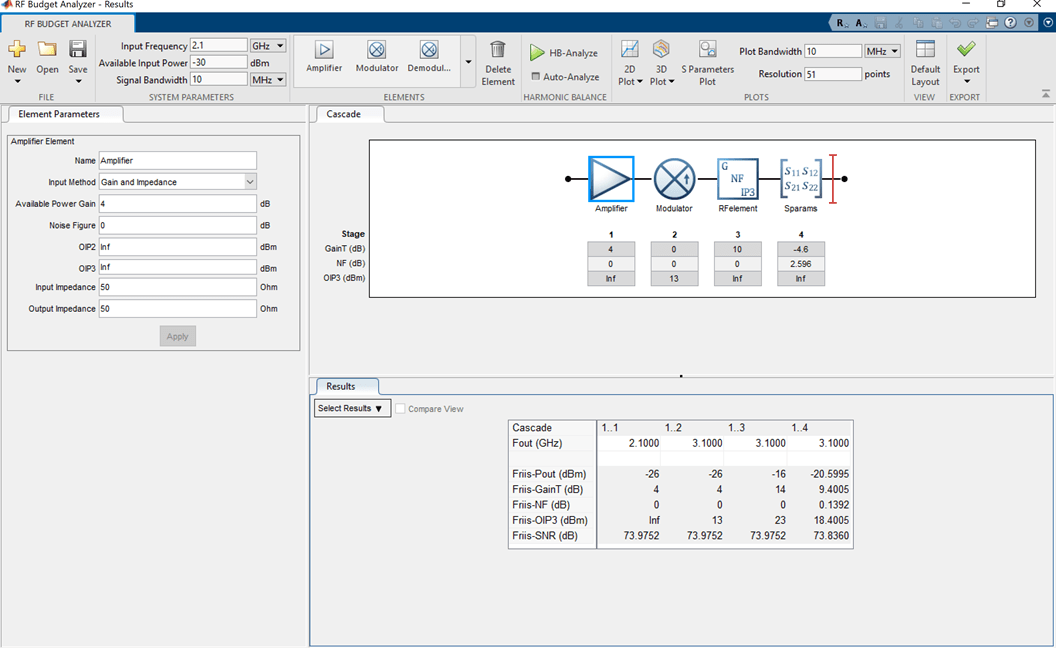# rfelement

Generic RF element object

## Description

Use the `rfelement` object to create a generic RF element. An RF element is a 2–port RF circuit object. You can use this element in the `rfbudget` object and the `circuit` object.

## Creation

### Syntax

``rfel = rfelement``
``rfel = rfelement(Name,Value)``

### Description

example

````rfel = rfelement` creates an RF element object with default property values. ```

example

````rfel = rfelement(Name,Value)` sets properties using one or more name-value pairs. You can specify multiple name-value pairs. Enclose each property name in a quote.```

## Properties

expand all

Name given to identify rf element, specified as a character vector. All names must be valid MATLAB® variable names.

Example: `'Name','rfel'`

Example: `rfel.Name = 'rfel'`

Available power gain, specified as a scalar in dB.

Example: `'Gain',10`

Example: `rfel.Gain = 10`

Noise figure, specified as a real finite nonnegative scalar dB.

Example: `'NF',-10`

Example: `rfel.NF = -10`

Output third-order intercept, specified as a scalar in dBm

Example: `'OIP3',10`

Example: `rfel.OIP3 = 10`

Input impedance, specified as a positive real part finite scalar in Ohms. You can also use a complex value with a positive real part.

Example: `'Zin',40`

Example: `rfel.Zin = 40`

Output impedance, specified as a scalar in Ohms. You can also use a complex value with a positive real part.

Example: `'Zout',40`

Example: `rfel.Zout = 40`

Number of ports, specified as a scalar integer. This property is read-only.

Names of port terminals, specified as a cell vector. This property is read-only.

## Examples

collapse all

Create an rfelement object with a gain of 10 dB, noise figure of 3 dB, and OIP3 (output third-order intercept) of 2 dBm.

`r = rfelement('Gain',10,'NF',3,'OIP3',2)`
```r = rfelement: RF element Name: 'RFelement' Gain: 10 NF: 3 OIP2: Inf OIP3: 2 Zin: 50 Zout: 50 NumPorts: 2 Terminals: {'p1+' 'p2+' 'p1-' 'p2-'} ```

Create an rf element with a gain of 4 dB. Create another rf element with an output third-order intercept(OIP3) of 13 dBm.

```rfel1 = rfelement('Gain',4); rfel2 = rfelement('OIP3',13);```

Build a 2-port circuit using the above defined rf elements.

`c = circuit([rfel1 rfel2])`
```c = circuit: Circuit element ElementNames: {'RFelement' 'RFelement_1'} Elements: [1x2 rfelement] Nodes: [0 1 2 3] Name: 'unnamed' NumPorts: 2 Terminals: {'p1+' 'p2+' 'p1-' 'p2-'} ```

Create an amplifier with a gain of 4 dB.

`a = amplifier('Gain',4);`

Create a modulator with an OIP3 of 13 dBm.

`m = modulator('OIP3',13);`

Create an nport using `passive.s2p`.

`n = nport('passive.s2p');`

Create an rf element with a gain of 10 dB.

`r = rfelement('Gain',10);`

Calculate the rf budget of a series of rf elements at an input frequency of 2.1 GHz, an available input power of -30 dBm, and a bandwidth of 10 MHz.

`b = rfbudget([a m r n],2.1e9,-30,10e6)`
```b = rfbudget with properties: Elements: [1x4 rf.internal.rfbudget.Element] InputFrequency: 2.1 GHz AvailableInputPower: -30 dBm SignalBandwidth: 10 MHz Solver: Friis AutoUpdate: true Analysis Results OutputFrequency: (GHz) [ 2.1 3.1 3.1 3.1] OutputPower: (dBm) [ -26 -26 -16 -20.6] TransducerGain: (dB) [ 4 4 14 9.4] NF: (dB) [ 0 0 0 0.1392] IIP2: (dBm) [] OIP2: (dBm) [] IIP3: (dBm) [ Inf 9 9 9] OIP3: (dBm) [ Inf 13 23 18.4] SNR: (dB) [73.98 73.98 73.98 73.84] ```

Show the analysis in the RF Budget Analyzer app.

`show(b)`